Скачать презентацию More on firm valuation Objective Discuss in

6c8224d3059cf3a78062d708003c806b.ppt

• Количество слайдов: 42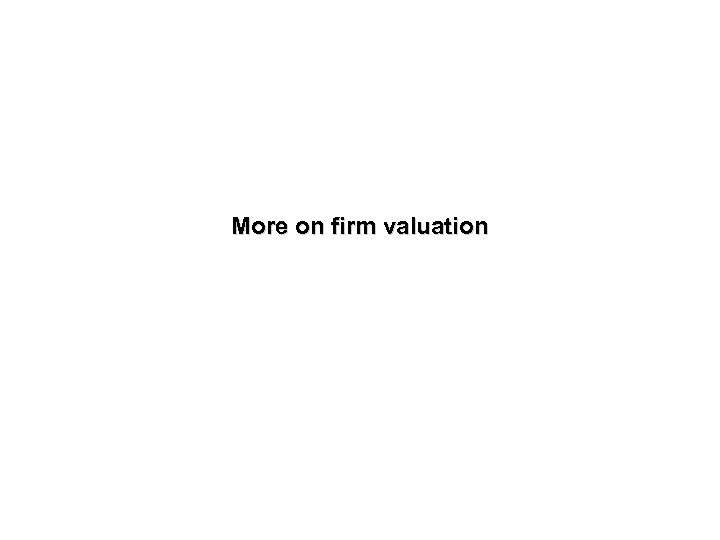More on firm valuation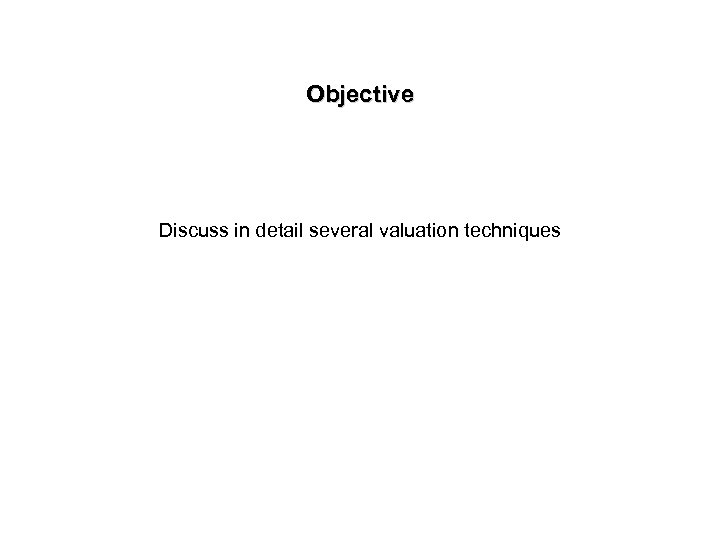Objective Discuss in detail several valuation techniques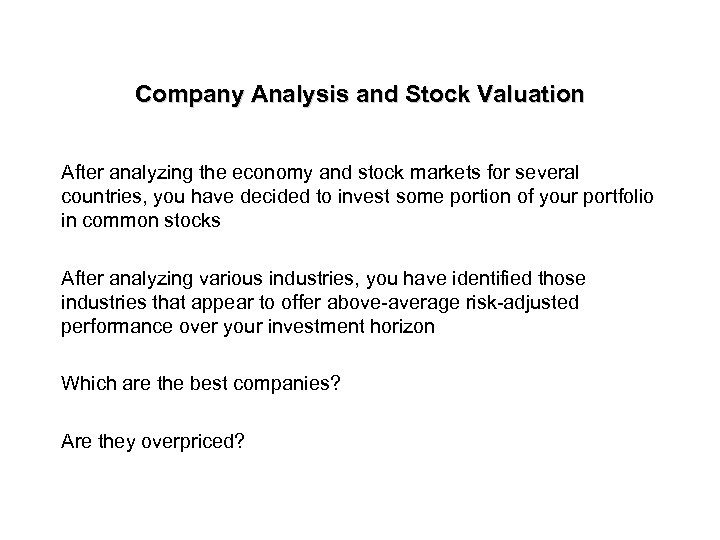Company Analysis and Stock Valuation After analyzing the economy and stock markets for several countries, you have decided to invest some portion of your portfolio in common stocks After analyzing various industries, you have identified those industries that appear to offer above-average risk-adjusted performance over your investment horizon Which are the best companies? Are they overpriced?Company Analysis and Stock Valuation • Industry competitive environment • SWOT analysis • Present value of cash flows • Relative valuation ratio techniques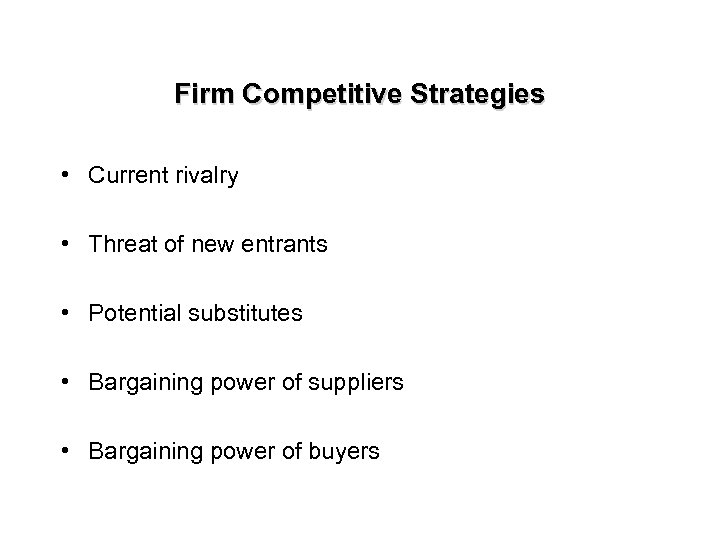Firm Competitive Strategies • Current rivalry • Threat of new entrants • Potential substitutes • Bargaining power of suppliers • Bargaining power of buyers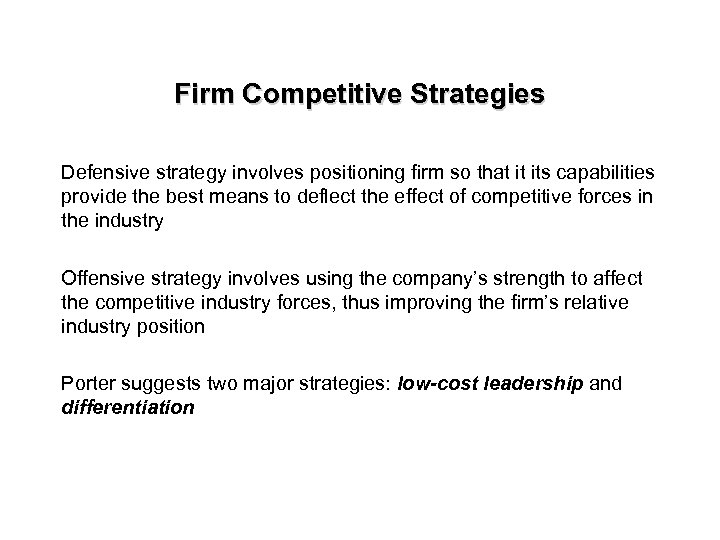Firm Competitive Strategies Defensive strategy involves positioning firm so that it its capabilities provide the best means to deflect the effect of competitive forces in the industry Offensive strategy involves using the company’s strength to affect the competitive industry forces, thus improving the firm’s relative industry position Porter suggests two major strategies: low-cost leadership and differentiation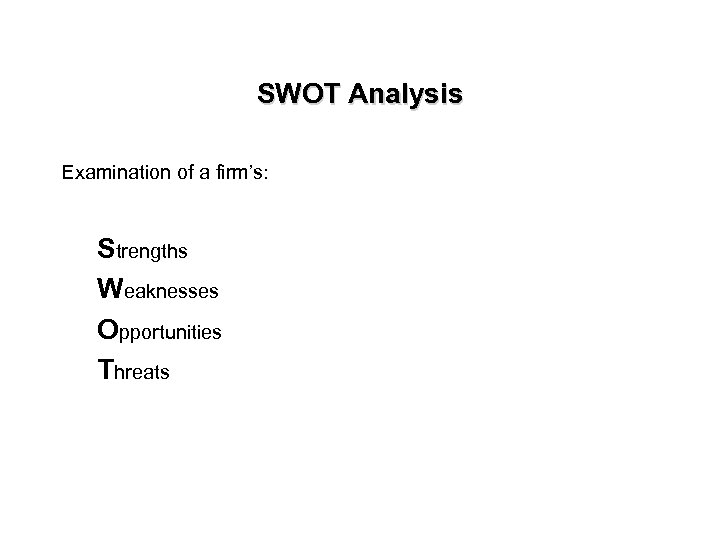SWOT Analysis Examination of a firm’s: Strengths Weaknesses Opportunities Threats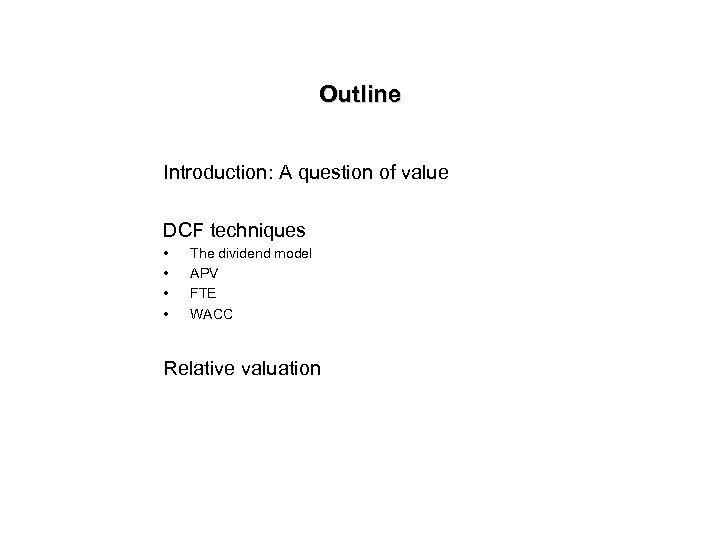Outline Introduction: A question of value DCF techniques • • The dividend model APV FTE WACC Relative valuation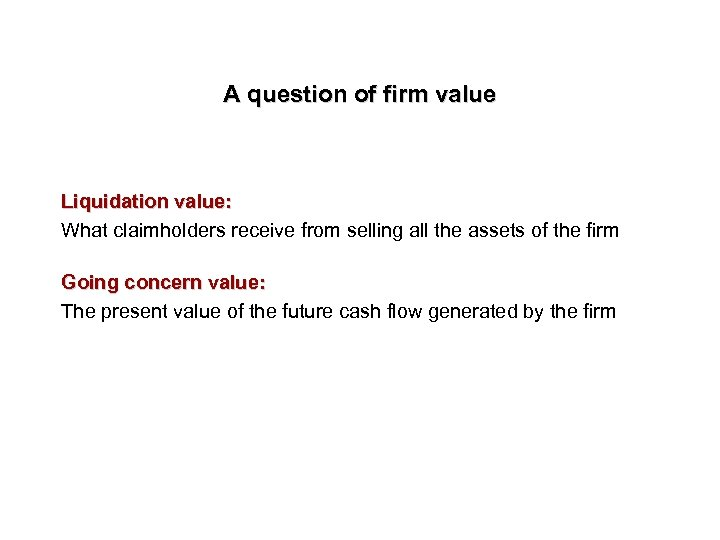A question of firm value Liquidation value: What claimholders receive from selling all the assets of the firm Going concern value: The present value of the future cash flow generated by the firm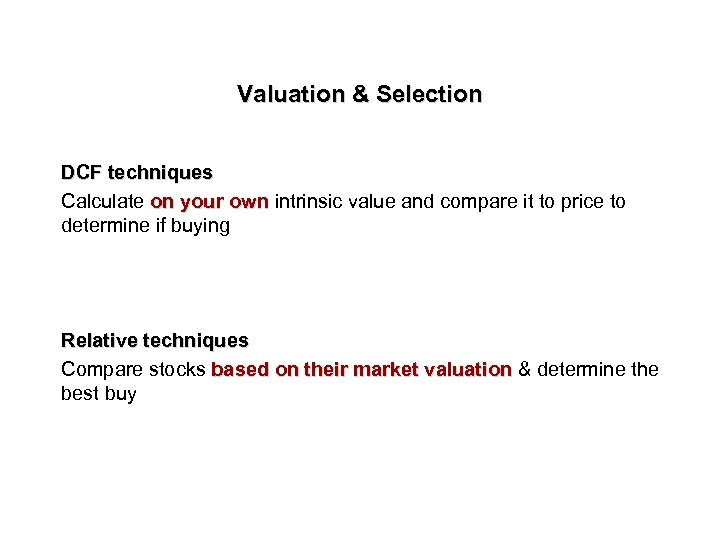Valuation & Selection DCF techniques Calculate on your own intrinsic value and compare it to price to own determine if buying Relative techniques Compare stocks based on their market valuation & determine the valuation best buy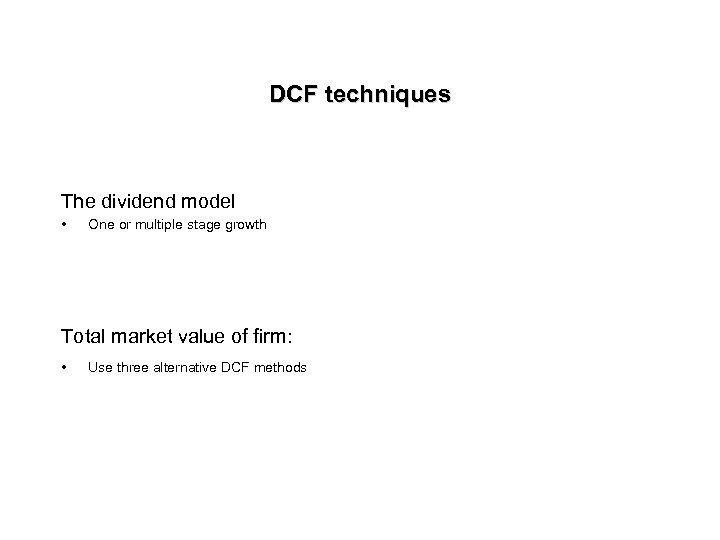DCF techniques The dividend model • One or multiple stage growth Total market value of firm: • Use three alternative DCF methods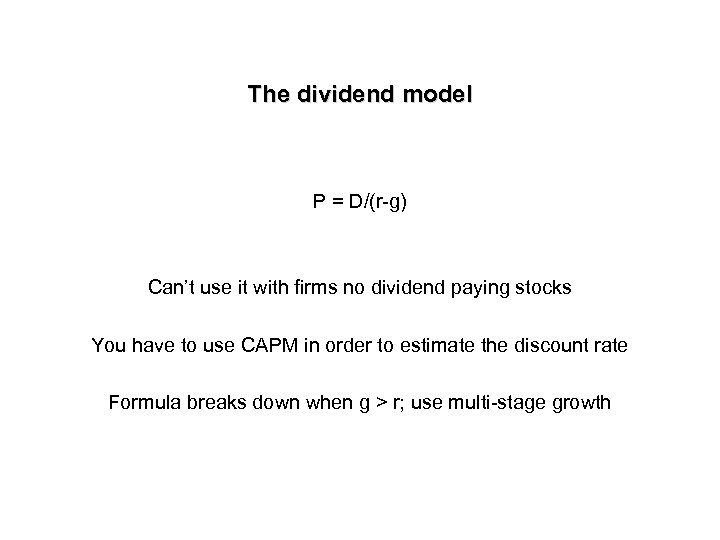The dividend model P = D/(r-g) Can’t use it with firms no dividend paying stocks You have to use CAPM in order to estimate the discount rate Formula breaks down when g > r; use multi-stage growth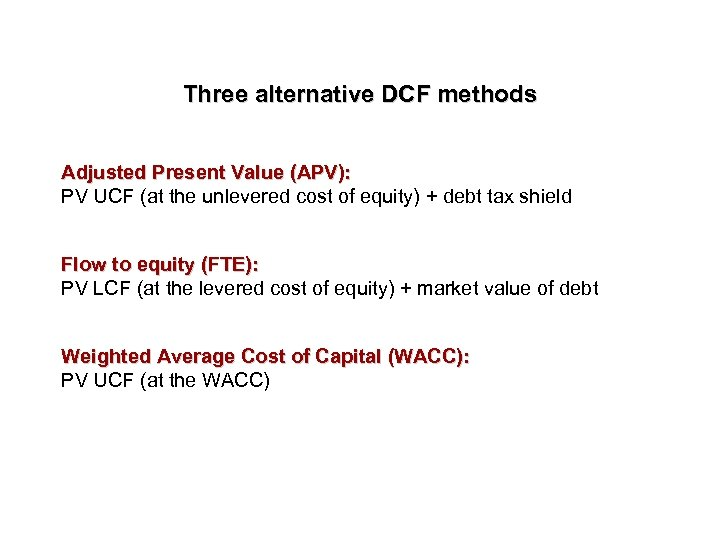Three alternative DCF methods Adjusted Present Value (APV): PV UCF (at the unlevered cost of equity) + debt tax shield Flow to equity (FTE): PV LCF (at the levered cost of equity) + market value of debt Weighted Average Cost of Capital (WACC): PV UCF (at the WACC)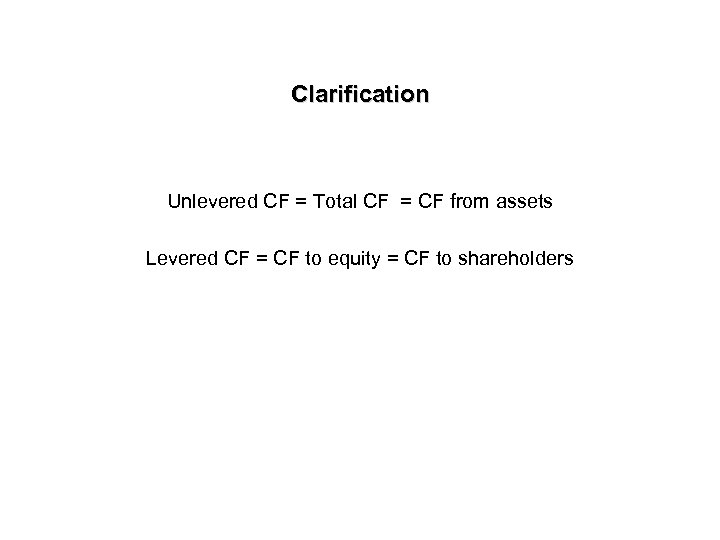Clarification Unlevered CF = Total CF = CF from assets Levered CF = CF to equity = CF to shareholders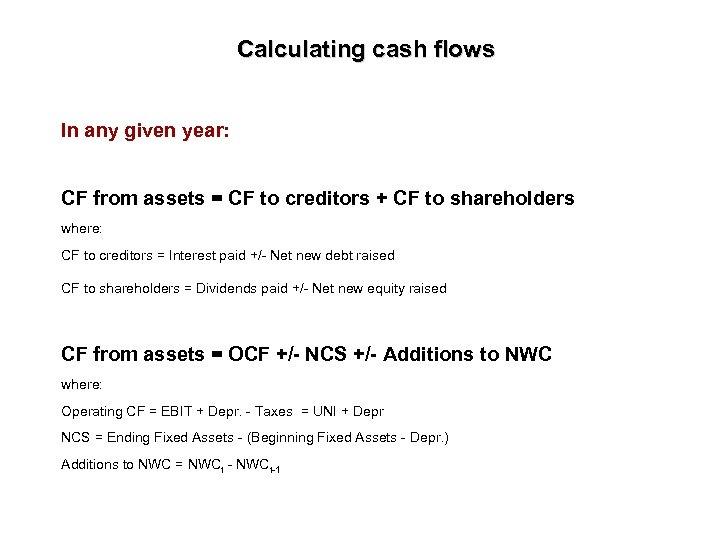Calculating cash flows In any given year: CF from assets = CF to creditors + CF to shareholders where: CF to creditors = Interest paid +/- Net new debt raised CF to shareholders = Dividends paid +/- Net new equity raised CF from assets = OCF +/- NCS +/- Additions to NWC where: Operating CF = EBIT + Depr. - Taxes = UNI + Depr NCS = Ending Fixed Assets - (Beginning Fixed Assets - Depr. ) Additions to NWC = NWCt - NWCt-1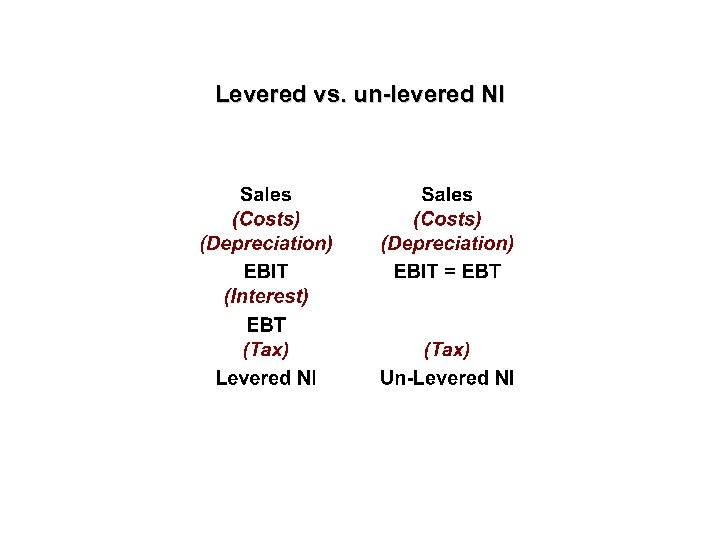Levered vs. un-levered NI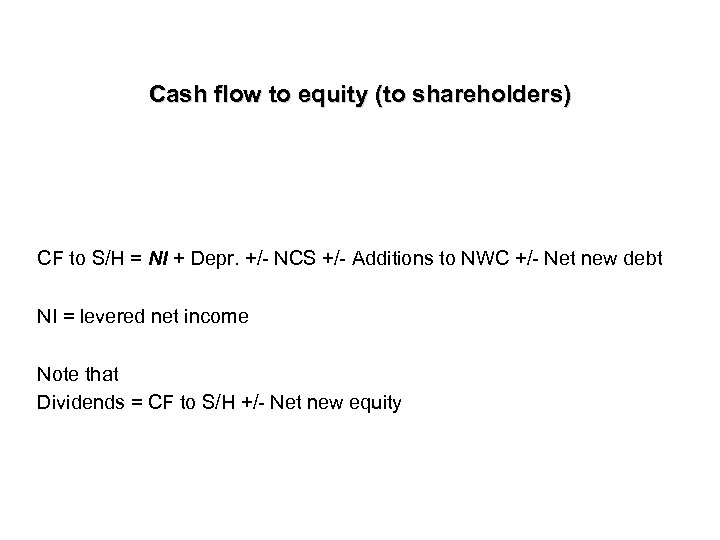Cash flow to equity (to shareholders) CF to S/H = NI + Depr. +/- NCS +/- Additions to NWC +/- Net new debt NI = levered net income Note that Dividends = CF to S/H +/- Net new equity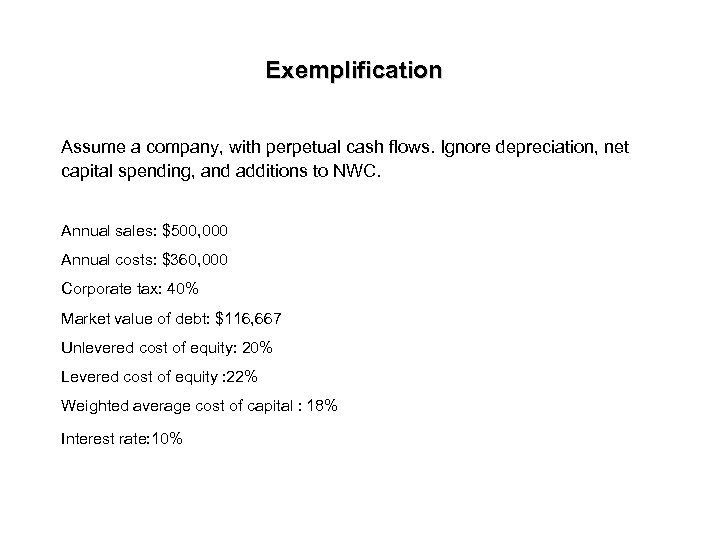Exemplification Assume a company, with perpetual cash flows. Ignore depreciation, net capital spending, and additions to NWC. Annual sales: \$500, 000 Annual costs: \$360, 000 Corporate tax: 40% Market value of debt: \$116, 667 Unlevered cost of equity: 20% Levered cost of equity : 22% Weighted average cost of capital : 18% Interest rate: 10%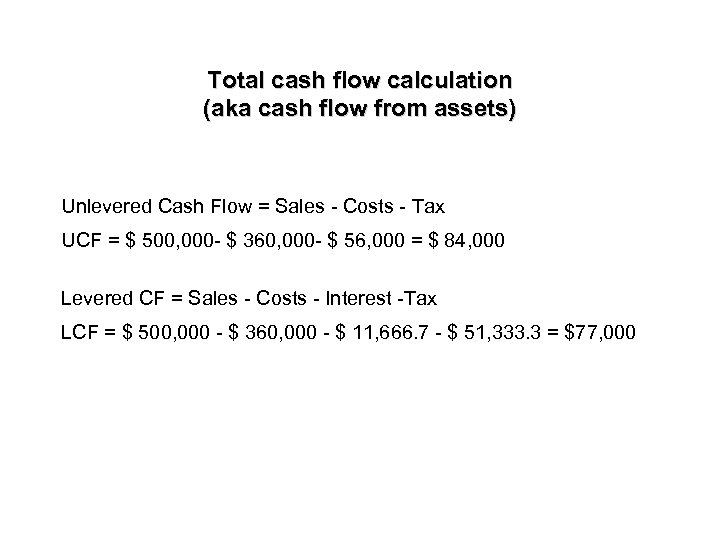Total cash flow calculation (aka cash flow from assets) Unlevered Cash Flow = Sales - Costs - Tax UCF = \$ 500, 000 - \$ 360, 000 - \$ 56, 000 = \$ 84, 000 Levered CF = Sales - Costs - Interest -Tax LCF = \$ 500, 000 - \$ 360, 000 - \$ 11, 666. 7 - \$ 51, 333. 3 = \$77, 000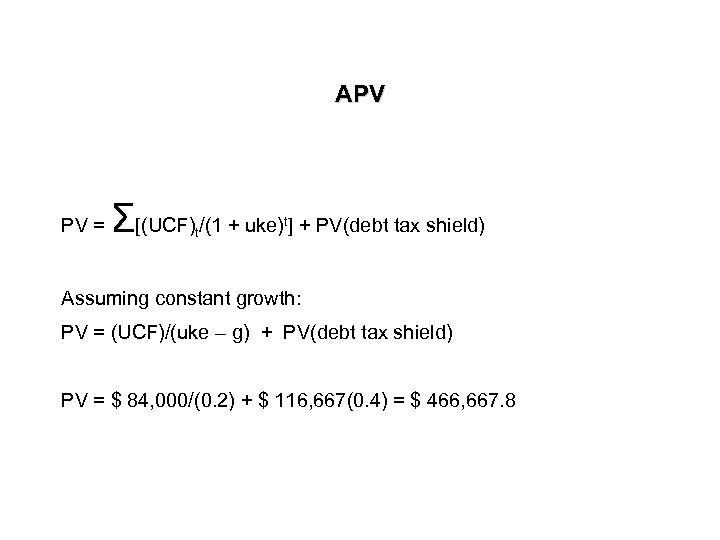APV PV = Σ[(UCF) /(1 + uke) ] + PV(debt tax shield) t t Assuming constant growth: PV = (UCF)/(uke – g) + PV(debt tax shield) PV = \$ 84, 000/(0. 2) + \$ 116, 667(0. 4) = \$ 466, 667. 8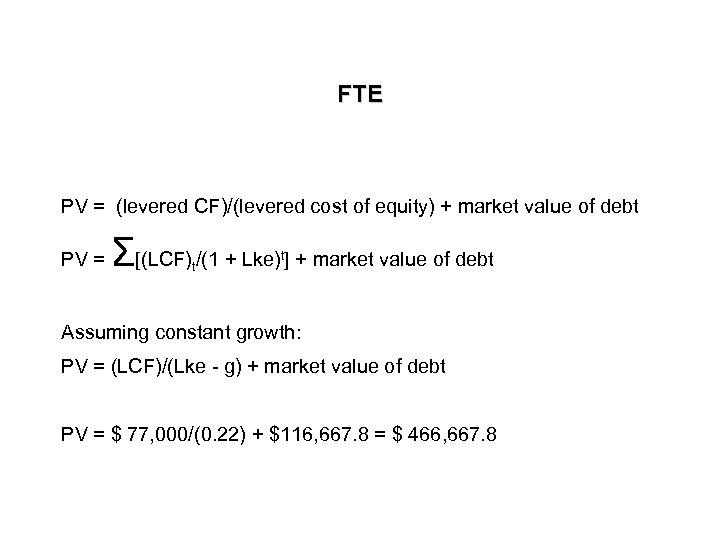FTE PV = (levered CF)/(levered cost of equity) + market value of debt PV = Σ[(LCF) /(1 + Lke) ] + market value of debt t t Assuming constant growth: PV = (LCF)/(Lke - g) + market value of debt PV = \$ 77, 000/(0. 22) + \$116, 667. 8 = \$ 466, 667. 8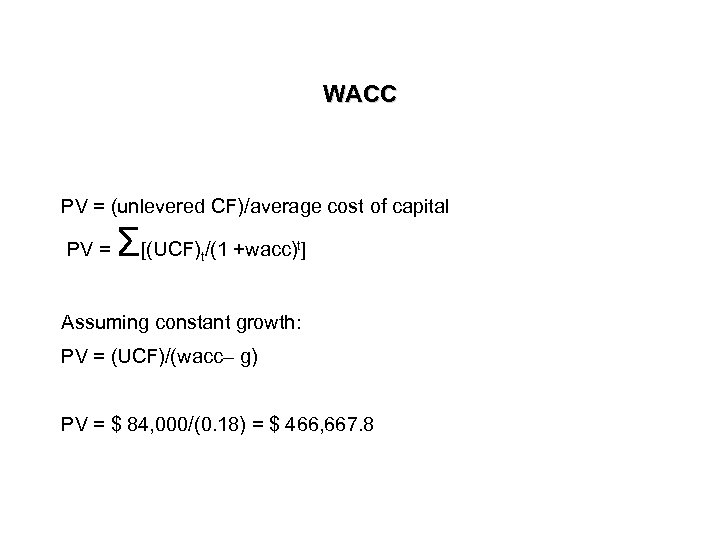WACC PV = (unlevered CF)/average cost of capital PV = Σ[(UCF) /(1 +wacc) ] t t Assuming constant growth: PV = (UCF)/(wacc– g) PV = \$ 84, 000/(0. 18) = \$ 466, 667. 8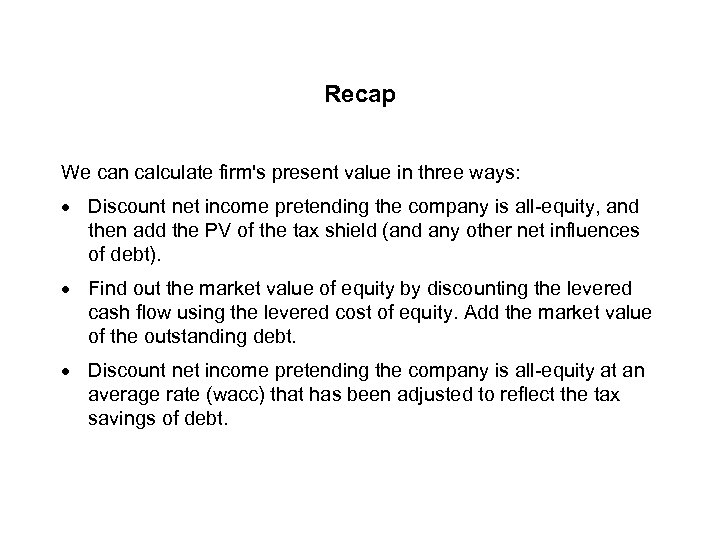Recap We can calculate firm's present value in three ways: · Discount net income pretending the company is all-equity, and then add the PV of the tax shield (and any other net influences of debt). · Find out the market value of equity by discounting the levered cash flow using the levered cost of equity. Add the market value of the outstanding debt. · Discount net income pretending the company is all-equity at an average rate (wacc) that has been adjusted to reflect the tax savings of debt.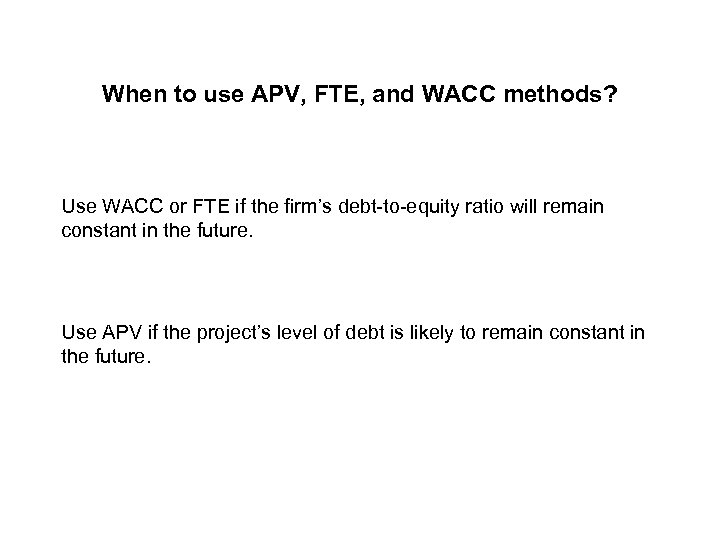When to use APV, FTE, and WACC methods? Use WACC or FTE if the firm’s debt-to-equity ratio will remain constant in the future. Use APV if the project’s level of debt is likely to remain constant in the future.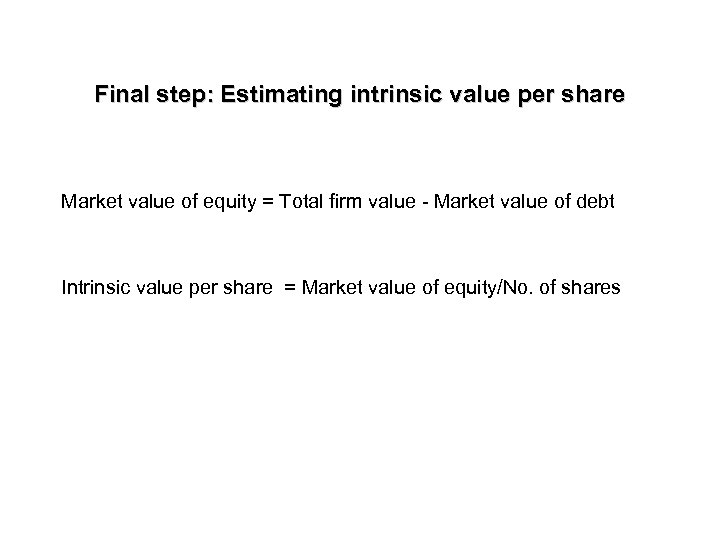Final step: Estimating intrinsic value per share Market value of equity = Total firm value - Market value of debt Intrinsic value per share = Market value of equity/No. of shares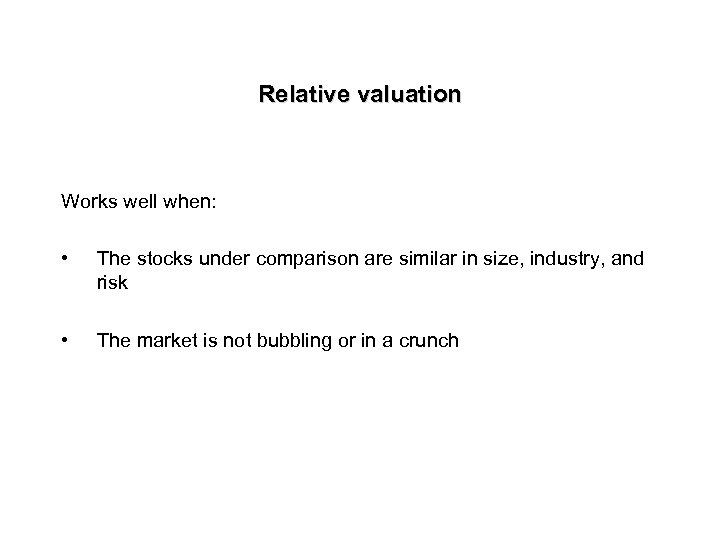Relative valuation Works well when: • The stocks under comparison are similar in size, industry, and risk • The market is not bubbling or in a crunch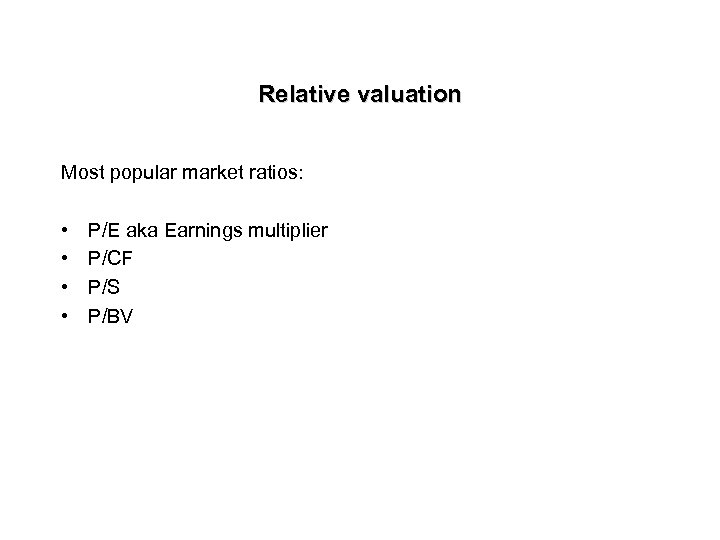Relative valuation Most popular market ratios: • • P/E aka Earnings multiplier P/CF P/S P/BV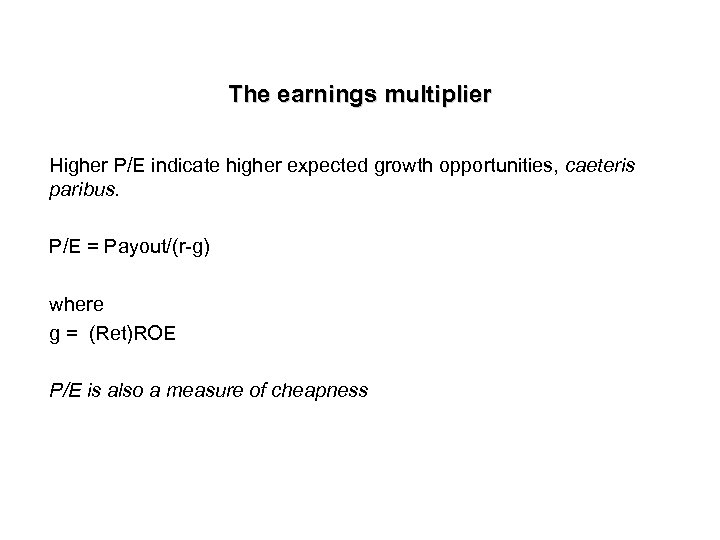The earnings multiplier Higher P/E indicate higher expected growth opportunities, caeteris paribus. P/E = Payout/(r-g) where g = (Ret)ROE P/E is also a measure of cheapness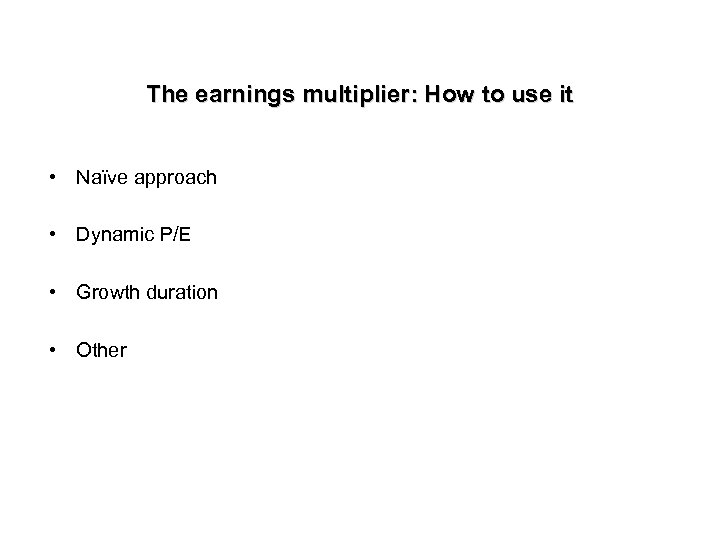The earnings multiplier: How to use it • Naïve approach • Dynamic P/E • Growth duration • Other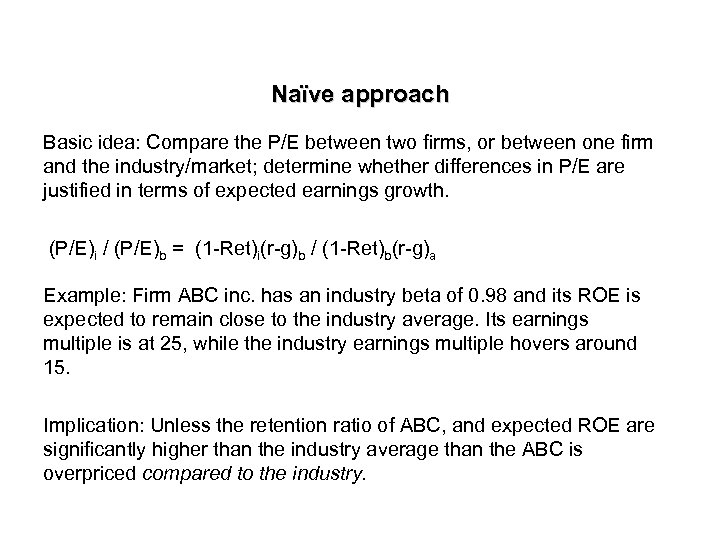Naïve approach Basic idea: Compare the P/E between two firms, or between one firm and the industry/market; determine whether differences in P/E are justified in terms of expected earnings growth. (P/E)i / (P/E)b = (1 -Ret)i(r-g)b / (1 -Ret)b(r-g)a Example: Firm ABC inc. has an industry beta of 0. 98 and its ROE is expected to remain close to the industry average. Its earnings multiple is at 25, while the industry earnings multiple hovers around 15. Implication: Unless the retention ratio of ABC, and expected ROE are significantly higher than the industry average than the ABC is overpriced compared to the industry.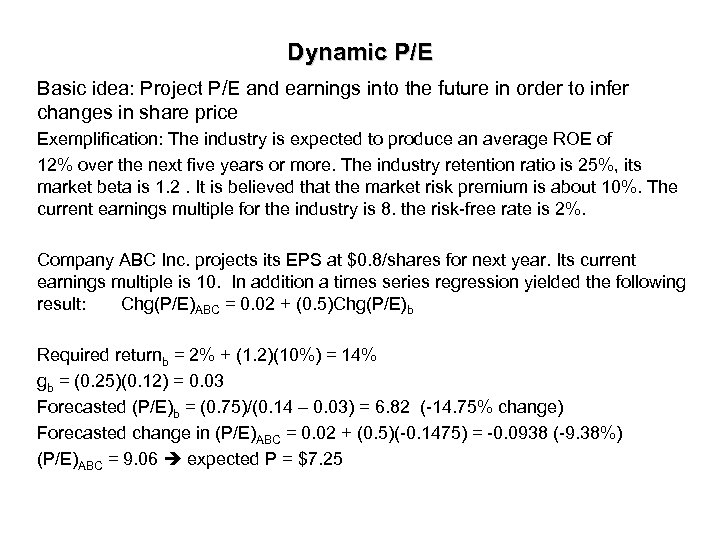Dynamic P/E Basic idea: Project P/E and earnings into the future in order to infer changes in share price Exemplification: The industry is expected to produce an average ROE of 12% over the next five years or more. The industry retention ratio is 25%, its market beta is 1. 2. It is believed that the market risk premium is about 10%. The current earnings multiple for the industry is 8. the risk-free rate is 2%. Company ABC Inc. projects its EPS at \$0. 8/shares for next year. Its current earnings multiple is 10. In addition a times series regression yielded the following result: Chg(P/E)ABC = 0. 02 + (0. 5)Chg(P/E)b Required returnb = 2% + (1. 2)(10%) = 14% gb = (0. 25)(0. 12) = 0. 03 Forecasted (P/E)b = (0. 75)/(0. 14 – 0. 03) = 6. 82 (-14. 75% change) Forecasted change in (P/E)ABC = 0. 02 + (0. 5)(-0. 1475) = -0. 0938 (-9. 38%) (P/E)ABC = 9. 06 expected P = \$7. 25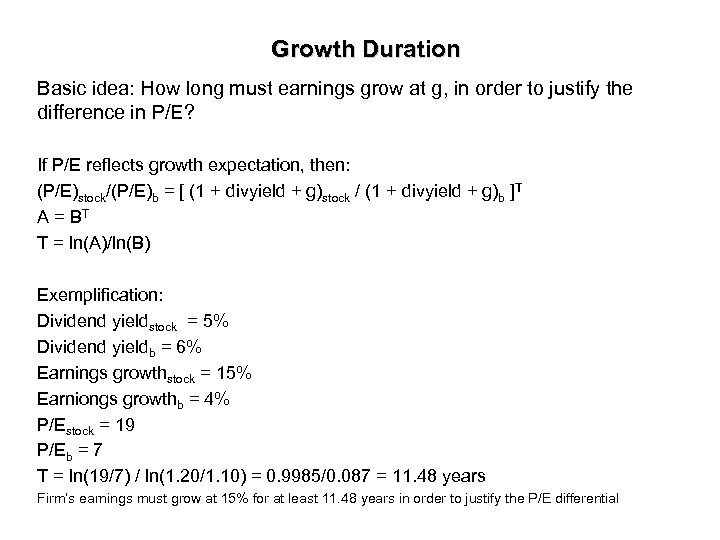Growth Duration Basic idea: How long must earnings grow at g, in order to justify the difference in P/E? If P/E reflects growth expectation, then: (P/E)stock/(P/E)b = [ (1 + divyield + g)stock / (1 + divyield + g)b ]T A = BT T = ln(A)/ln(B) Exemplification: Dividend yieldstock = 5% Dividend yieldb = 6% Earnings growthstock = 15% Earniongs growthb = 4% P/Estock = 19 P/Eb = 7 T = ln(19/7) / ln(1. 20/1. 10) = 0. 9985/0. 087 = 11. 48 years Firm’s earnings must grow at 15% for at least 11. 48 years in order to justify the P/E differential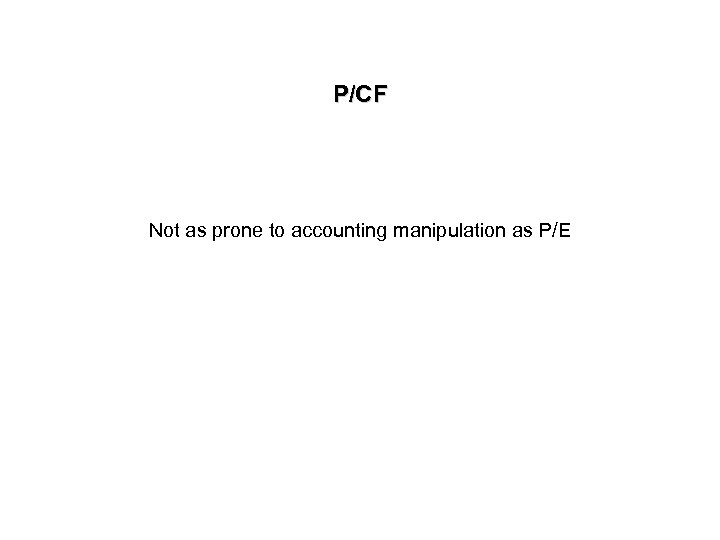P/CF Not as prone to accounting manipulation as P/E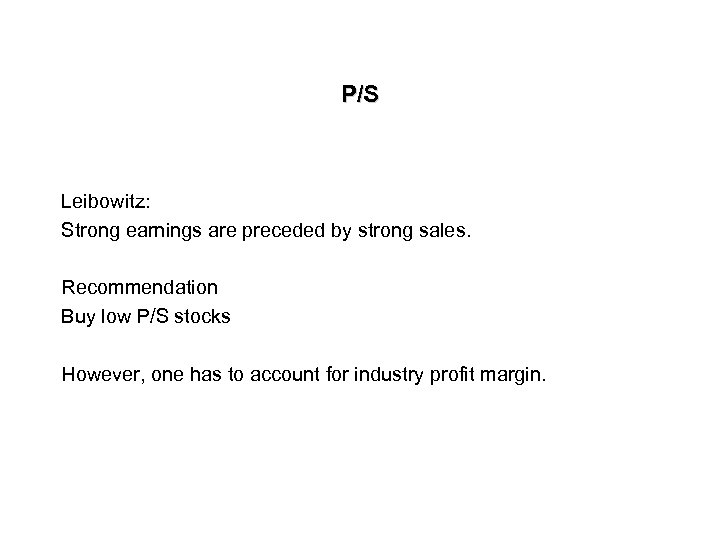P/S Leibowitz: Strong earnings are preceded by strong sales. Recommendation Buy low P/S stocks However, one has to account for industry profit margin.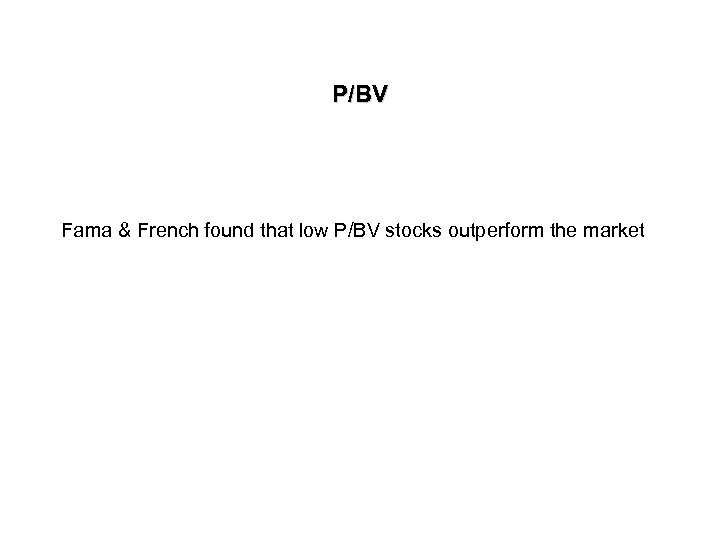P/BV Fama & French found that low P/BV stocks outperform the market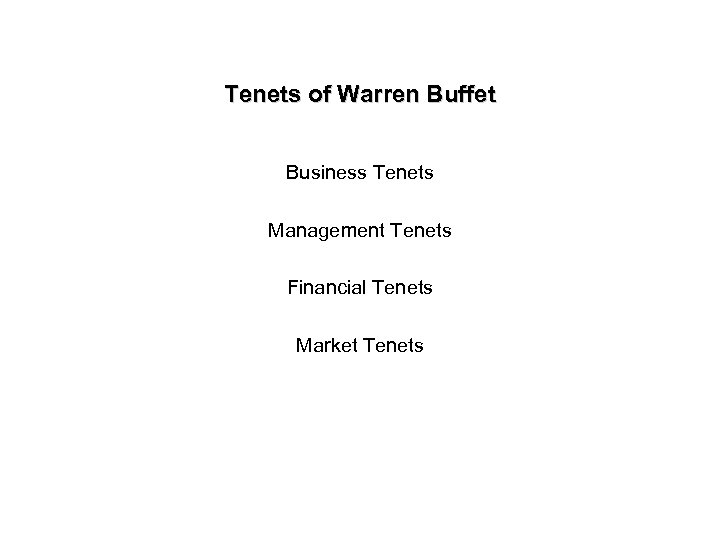Tenets of Warren Buffet Business Tenets Management Tenets Financial Tenets Market Tenets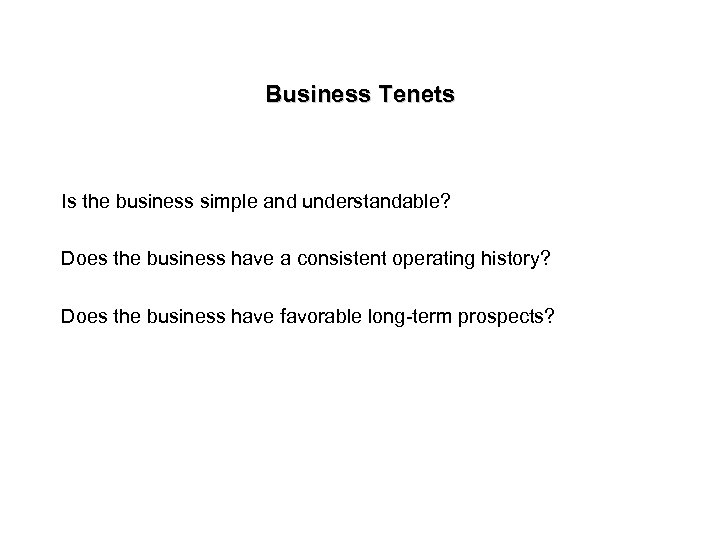Business Tenets Is the business simple and understandable? Does the business have a consistent operating history? Does the business have favorable long-term prospects?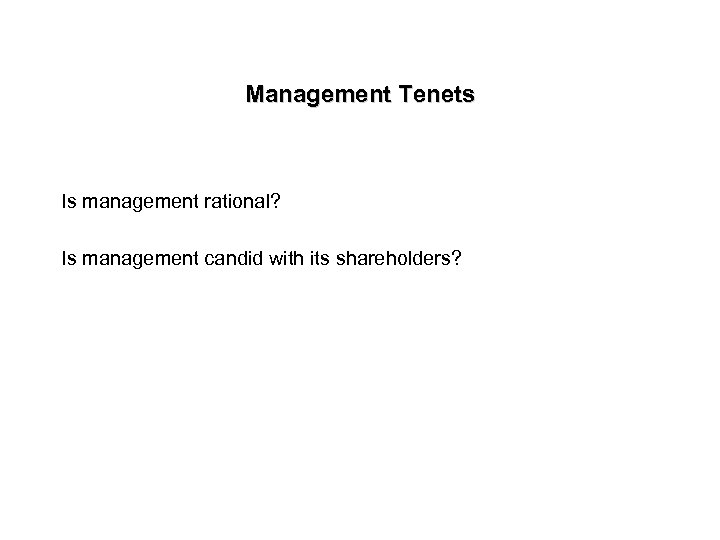Management Tenets Is management rational? Is management candid with its shareholders?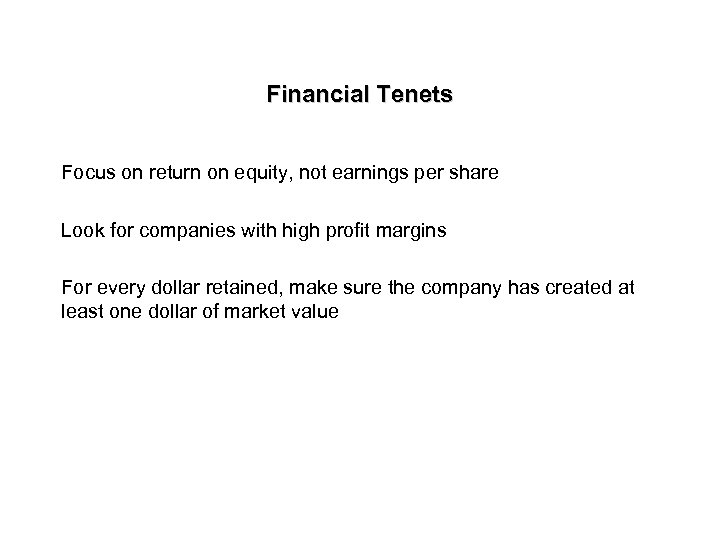Financial Tenets Focus on return on equity, not earnings per share Look for companies with high profit margins For every dollar retained, make sure the company has created at least one dollar of market value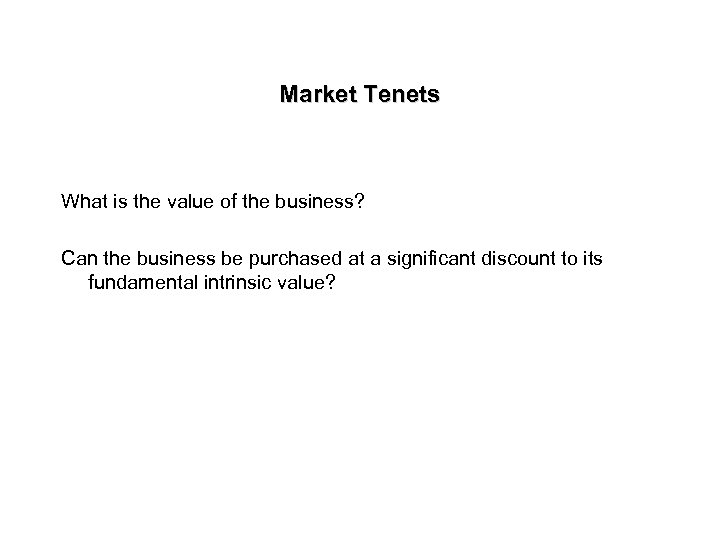Market Tenets What is the value of the business? Can the business be purchased at a significant discount to its fundamental intrinsic value?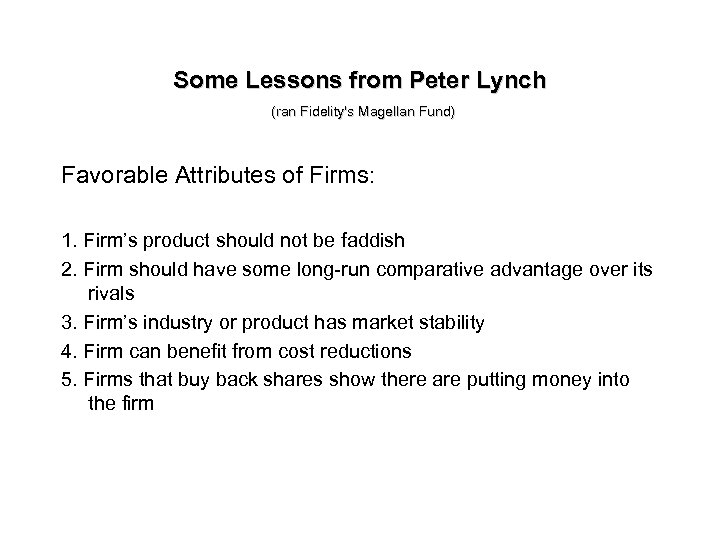Some Lessons from Peter Lynch (ran Fidelity's Magellan Fund) Favorable Attributes of Firms: 1. Firm’s product should not be faddish 2. Firm should have some long-run comparative advantage over its rivals 3. Firm’s industry or product has market stability 4. Firm can benefit from cost reductions 5. Firms that buy back shares show there are putting money into the firm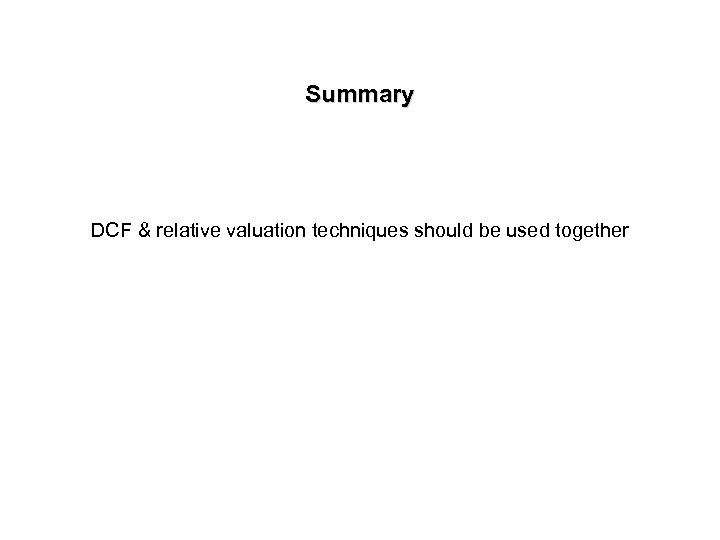Summary DCF & relative valuation techniques should be used together